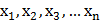# Tangent is drawn at the pointon the curve, which intersects the-axis at. Now, again a tangent is drawn aton the curve which intersect the-axis atand the process is repeatedtimes, i.e.,. Ifform an arithmetic progression with common difference equal toand curve passes through (0, 2). Now if curve passes through the point, then the value ofis

## Question ID - 153326 :- Tangent is drawn at the pointon the curve, which intersects the-axis at. Now, again a tangent is drawn aton the curve which intersect the-axis atand the process is repeatedtimes, i.e.,. Ifform an arithmetic progression with common difference equal toand curve passes through (0, 2). Now if curve passes through the point, then the value ofis

3537

 (8) Equation of tangent atof(1) This tangent cuts the-axis soare in APgivenIntegrating both sidespasses through (0,2)